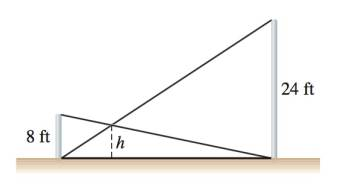Chapter A, Problem 35E### Algebra and Trigonometry (MindTap ...

4th Edition
James Stewart + 2 others
ISBN: 9781305071742

#### Solutions

Chapter
Section### Algebra and Trigonometry (MindTap ...

4th Edition
James Stewart + 2 others
ISBN: 9781305071742
Textbook Problem

# Finding a Length Two vertical poles, one 8 ft tall and the other 24 ft tall, have ropes stretched from the top of each to the base of the other (see the figure). How high above the ground is the point where the ropes cross? [Hint: Use similarity]To determine

The height of the point above the ground where the ropes cross.

Explanation

Given:

One pole is 8 ft tall and other pole is 24 ft tall.

Both have ropes stretched from the top of each to the base of the other.

Figure (1)

Approach:

If all three angles of a triangle are equal to corresponding angles of the other triangle, then both the triangles are similar and the rule is referred to as AAA rule.

Calculation:

By AAA rule, triangle AEF is similar to triangle ADC.

So,

AEh=AE+EDDC=AE+ED24 …(1)

By AAA Rule, triangle BAD is similar to triangle FED.

So,

EDh=AE+EDAB=AE+ED8

AE+ED=8EDh(2)

Substitute value of AE+ED from equation (2) in equation (1)

### Still sussing out bartleby?

Check out a sample textbook solution.

See a sample solution

#### The Solution to Your Study Problems

Bartleby provides explanations to thousands of textbook problems written by our experts, many with advanced degrees!

Get Started